The Trouble with Typing Type as Type
10th September 2015
12:00
liamoc.net

Axiomatic set theories such as that of Zermelo and Fraenkel, in their attempt to provide a comprehensive foundation for mathematics, involve several intricate tricks to avoid becoming inconsistent. A suitably naïve set theory is already inconsistent due to the infamous paradoxical set of Russell (1938).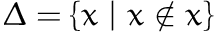Here we have used set comprehension to define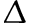as the set of all sets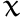that do not contain themselves. This leads to the question, doescontain? Ifis an element of, then it is not, asonly contains sets that do not contain themselves. Ifis not an element of, then it is, asdoes not contain itself – We have a paradox!

To address this, different foundations take different approaches. Most axiomatic set theories eliminate or restrict the rule of comprehension, that is, they don’t allow sets to be constructed from arbitrary predicates. Instead, set comprehension can only be used to describe subsets of already constructed sets. This prevents comprehension from being used above, but it also prevents a lot of other useful constructions, like products or unions! Thus a handful of other axioms to construct sets are added, such as pairing, union, powerset and so on (all nicely explained in Halmos (1960)).

Another axiom, that of regularity, says1 that there is no infinite sequence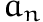such that, for any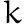,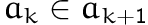. This implies that no set can contain itself, and allows us to build the universe of set theory by stages, called ranks. At rank zero, no sets exist; at rank one, there is just the empty set; at rank two, there is also the set containing the empty set; and at each following rank, the added sets all contain the sets that are defined at earlier ranks, as shown in the following figure: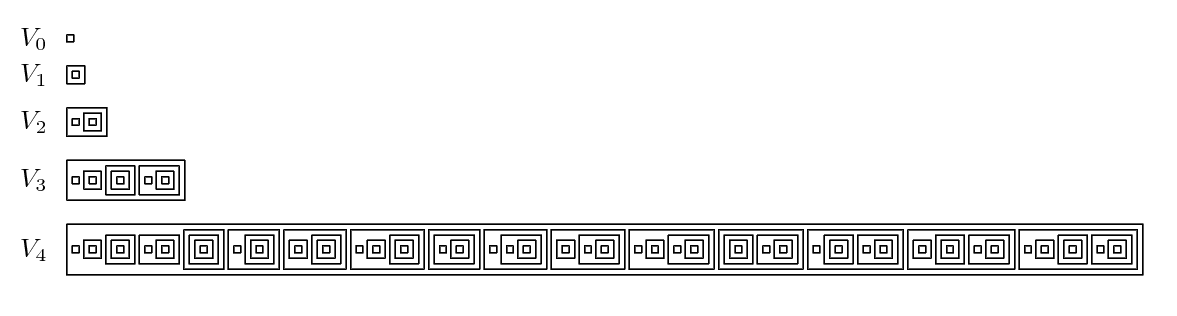The entire universe of set theory can be thought of as the union of the universe at each rank,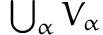, a presentation originally due to Zermelo (1930), but commonly attributed to John von Neumann.

## Moving to Type Theory

This stratification bears remarkable similarity to Russell’s theory of types (see Russell (1938)), his own solution to the the paradoxical set, and the distant ancestor of modern type theory.

Indeed, in the intuitionistic type theory of Martin-Löf (1984), the approximate foundation of the Agda proof assistant, we have a heirarchy of types that very much resembles that of von Neumann or Zermelo2: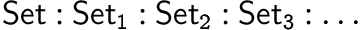The rule of cumulativity, which is not present in Agda3, but exists in some type theories and languages such as Idris, makes this resemblance even stronger:This rule implies that like the von Neumann rank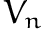, a type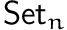is inhabited by every type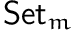where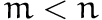.

The differences between the two theories start to emerge when one examines why this stratification exists in type theory. In axiomatic set theory, eliminating the axiom of regularity and thus the stratification it implies makes it rather difficult to do induction, but it does not make the theory inconsistent – there have been several non-well-founded set theories proposed, such the hyperset theory of Aczel (1988), which do exactly this.

Removing unrestricted set comprehension is enough to avoid Russell’s paradox, as it allows us to distinguish between formulae (or predicates) and sets. Unlike informal set theory, we cannot construct a set for any given formula. For example,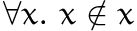is a valid formula, but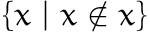is not a set.

Type theories are not set theories – they do not have a separate logical formula language, like that of Frege, to serve as a basis for the theory. So, one cannot achieve consistency in type theory by restricting how a set may be constructed from a logical formula. Instead, type theory places restrictions on the kinds of formulae that can be expressed. Rather that rule out paradoxical sets representing self-referential propositions, type theory rules out the propositions themselves. In such a theory, it is not even well-formed to ask if a set contains itself4.

This restriction is a consequence of the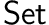hierarchy mentioned earlier – remove this from type theory, by saying instead that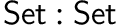, and the result is more or less equivalent to Falso5. We can show that type theory is inconsistent with this change using Girard’s paradox, which is a generalised encoding of Russell’s paradox for pure type systems. The contradiction derived from this paradox is rather involved, so much so that Martin-Löf himself didn’t realise that it applied to the first version of his type theory. Hurkens (1995) provided a simplification, which is encoded in Agda here.

With inductive types, however, we can use Russell’s paradox directly, by formalising a naïve notion of sets as comprehensions, and using this to derive a contradiction.

For these (interactive) Agda snippets, I have enabled --type-in-type, which removes the predicative heirarchy from the type theory, instead stating that.

data SET : Set where
set : (X : Set) → (X → SET) → SET


This defines a set (written set X f) as a comprehension over an carrier type X and a function f, where the element for a given index value x : X is given by f x. This definition is already using the fact that– normally, a type (of type) would not be permitted as a parameter to set, which constructs a type of the same size.

The empty set, having no elements, uses the empty type as its carrier :

∅ : SET
∅ = set ⊥ ⊥-elim


The set containing the empty set, having one element, uses the unit type as its carrier:

⟨∅⟩ : SET
⟨∅⟩ = set ⊤ (λ _ → ∅)


The next rank,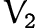, has two elements, and thus can use Bool as its carrier:

⟨∅,⟨∅⟩⟩ : SET
⟨∅,⟨∅⟩⟩ = set Bool (λ x → if x then ∅ else ⟨∅⟩)


More sets could be defined using similar techniques, so I will forgo any further definitions.

We can also define the membership operators for our SET type:

_∈_ : SET → SET → Set
a ∈ set X f = Σ X (λ x → a ≡ f x)

_∉_ : SET → SET → Set
a ∉ b = (a ∈ b) → ⊥


A value of type a ∈ set X f can be thought of as a proof that there exists a value x : X for which the element function f gives a.

Using these operators, we can define Russell’s paradoxical setas follows:

Δ : SET
Δ = set (Σ SET (λ s → s ∉ s)) proj₁


This is a set which, for its carrier type, uses pairs containing a set s and a proof that s does not contain itself. The element function just discards the proof, leaving us with the SET of all SETs that do not contain themselves.

Indeed, we can prove that every set which is in Δ does not contain itself:

x∈Δ→x∉x : ∀ {X} → X ∈ Δ → X ∉ X
x∈Δ→x∉x ((Y , Y∉Y) , refl) = Y∉Y

A corollary of this is that Δ itself does not contain itself:
Δ∉Δ : Δ ∉ Δ
Δ∉Δ Δ∈Δ = x∈Δ→x∉x Δ∈Δ Δ∈Δ


But we know that every set which does not contain itself is in Δ:

x∉x→x∈Δ : ∀ {X} →  X ∉ X → X ∈ Δ
x∉x→x∈Δ {X} X∉X = (X , X∉X) , refl


And from this we can derive a contradiction:

falso : ⊥
falso = Δ∉Δ (x∉x→x∈Δ Δ∉Δ)


## Musings and Speculation

I find it very curious that two very different approaches to formalising mathematics end up with much the same stratified character, and for different reasons. Perhaps this Russell-style heirarchy is, kind of like the Church-Turing thesis, a fundamental characteristic of any sufficiently expressive foundation. Something discovered rather than invented. In the words of Scott (1974):

The truth is that there is only one satisfactory way of avoiding the paradoxes: namely, the use of some form of the theory of types. That was at the basis of both Russell’s and Zermelo’s intuitions. Indeed the best way to regard Zermelo’s theory is as a simplification and extension of Russell’s. (We mean Russell’s simple theory of types, of course.) The simplification was to make the types cumulative. Thus mixing of types is easier and annoying repetitions are avoided. Once the later types are allowed to accumulate the earlier ones, we can then easily imagine extending the types into the transfinite – just how far we want to go must necessarily be left open. Now Russell made his types explicit in his notation and Zermelo left them implicit. [emphasis in original]

## Acknowledgements

The Agda development in this post is taken from one of Thorsten Altenkirch’s lectures, the code of which is available here. The original proof is, as far as I can tell, due to Chad E Brown, who formulated the same thing in Coq.

Aczel, P. (1988). Non-well-founded sets, Center for the Study of Language; Information, Stanford University.
Halmos, P. (1960). Naïve Set Theory, Van Nostrand.
Hurkens, A. J. C. (1995). A simplification of Girard’s Paradox. In M. Dezani-Ciancaglini & G. Plotkin, eds., Typed lambda calculi and applications: Proceedings of the 2nd international conference on typed lambda calculi and applications (TLCA-95), Berlin, Heidelberg: Springer, pp. 266–278.
Martin-Löf, P. (1984). Intuitionistic Type Theory, Bibliopolis.
Russell, B. (1938). Principles of Mathematics, 2nd edn, Vol. 1, W.W. Norton.
Scott, D. (1974). Axiomatizing set theory. In Axiomatic set theory (Proceedings of the Symposium on Pure Mathematics, Vol. XIII, Part II, University of California, Los Angeles, California, 1967), American Mathematics Society, Providence, R.I., pp. 207–214.
Zermelo, E. (1930). Über grenzzahlen und mengenbereiche: Neue untersuchungen über die grundlagen der mengenlehre. Fundamenta Mathematicae, 16, 29–47.

1. This presentation is not the normal one found in textbooks, which is that every non-empty set contains an element that is disjoint from itself, but that presentation is more brain-bending, and is implied by the statement presented here if you include the axiom of dependent choice.↩︎

2. Here,is the type given to types, similar to the kind * in Haskell, and is not a reference to the sets of axiomatic set theory.↩︎

3. Agda makes use of explicit universe polymorphism instead, and I’m still undecided which version of type theory I like better.↩︎

4. In set theory, it’s a valid question to ask, just the answer is always “no.”↩︎